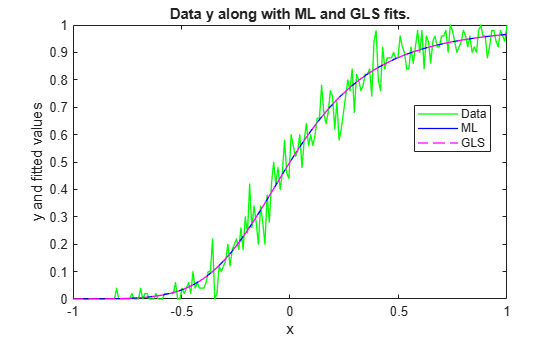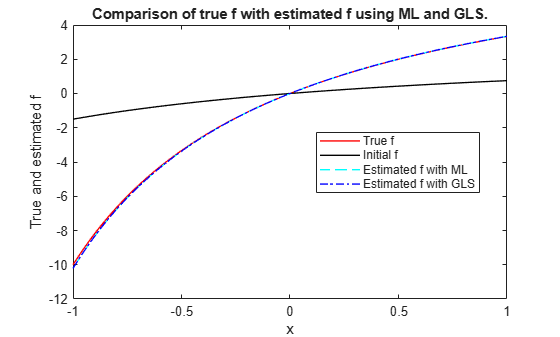# Nonlinear Logistic Regression

This example shows two ways of fitting a nonlinear logistic regression model. The first method uses maximum likelihood (ML) and the second method uses generalized least squares (GLS) via the function fitnlm from Statistics and Machine Learning Toolbox (TM).

### Problem Description

Logistic regression is a special type of regression in which the goal is to model the probability of something as a function of other variables. Consider a set of predictor vectorswhereis the number of observations andis a column vector containing the values of thepredictors for theth observation. The response variable foriswhererepresents a Binomial random variable with parameters, the number of trials, and, the probability of success for trial. The normalized response variable is- the proportion of successes intrials for observation. Assume that responsesare independent for. For each:.

Consider modelingas a function of predictor variables.

In linear logistic regression, you can use the function fitglm to modelas a function ofas follows:withrepresenting a set of coefficients multiplying the predictors in. However, suppose you need a nonlinear function on the right-hand-side:There are functions in Statistics and Machine Learning Toolbox (TM) for fitting nonlinear regression models, but not for fitting nonlinear logistic regression models. This example shows how you can use toolbox functions to fit those models.

### Direct Maximum Likelihood (ML)

The ML approach maximizes the log likelihood of the observed data. The likelihood is easily computed using the Binomial probability (or density) function as computed by the binopdf function.

### Generalized Least Squares (GLS)

You can estimate a nonlinear logistic regression model using the function fitnlm. This might seem surprising at first since fitnlm does not accommodate Binomial distribution or any link functions. However, fitnlm can use Generalized Least Squares (GLS) for model estimation if you specify the mean and variance of the response. If GLS converges, then it solves the same set of nonlinear equations for estimatingas solved by ML. You can also use GLS for quasi-likelihood estimation of generalized linear models. In other words, we should get the same or equivalent solutions from GLS and ML. To implement GLS estimation, provide the nonlinear function to fit, and the variance function for the Binomial distribution.

Mean or model function

The model function describes howchanges with. For fitnlm, the model function is:Weight function

fitnlm accepts observation weights as a function handle using the 'Weights' name-value pair argument. When using this option, fitnlm assumes the following model:where responsesare assumed to be independent, andis a custom function handle that acceptsand returns an observation weight. In other words, the weights are inversely proportional to the response variance. For the Binomial distribution used in the logistic regression model, create the weight function as follows:fitnlm models the variance of the responseaswhereis an extra parameter that is present in GLS estimation, but absent in the logistic regression model. However, this typically does not affect the estimation of, and it provides a "dispersion parameter" to check on the assumption that thevalues have a Binomial distribution.

An advantage of using fitnlm over direct ML is that you can perform hypothesis tests and compute confidence intervals on the model coefficients.

### Generate Example Data

To illustrate the differences between ML and GLS fitting, generate some example data. Assume thatis one dimensional and suppose the true functionin the nonlinear logistic regression model is the Michaelis-Menten model parameterized by avector:myf = @(beta,x) beta(1)*x./(beta(2) + x); 

Create a model function that specifies the relationship betweenand.

 mymodelfun = @(beta,x) 1./(1 + exp(-myf(beta,x))); 

Create a vector of one dimensional predictors and the true coefficient vector.

 rng(300,'twister'); x = linspace(-1,1,200)'; beta = [10;2]; 

Compute a vector ofvalues for each predictor.

 mu = mymodelfun(beta,x); 

Generate responsesfrom a Binomial distribution with success probabilitiesand number of trials.

 n = 50; z = binornd(n,mu); 

Normalize the responses.

 y = z./n; 

### ML Approach

The ML approach defines the negative log likelihood as a function of thevector, and then minimizes it with an optimization function such as fminsearch. Specify beta0 as the starting value for.

 mynegloglik = @(beta) -sum(log(binopdf(z,n,mymodelfun(beta,x)))); beta0 = [3;3]; opts = optimset('fminsearch'); opts.MaxFunEvals = Inf; opts.MaxIter = 10000; betaHatML = fminsearch(mynegloglik,beta0,opts) 
betaHatML = 9.9259 1.9720 

The estimated coefficients in betaHatML are close to the true values of [10;2].

### GLS Approach

The GLS approach creates a weight function for fitnlm previously described.

 wfun = @(xx) n./(xx.*(1-xx)); 

Call fitnlm with custom mean and weight functions. Specify beta0 as the starting value for.

 nlm = fitnlm(x,y,mymodelfun,beta0,'Weights',wfun) 
nlm = Nonlinear regression model: y ~ F(beta,x) Estimated Coefficients: Estimate SE tStat pValue ________ _______ ______ __________ b1 9.926 0.83135 11.94 4.193e-25 b2 1.972 0.16438 11.996 2.8182e-25 Number of observations: 200, Error degrees of freedom: 198 Root Mean Squared Error: 1.16 R-Squared: 0.995, Adjusted R-Squared 0.995 F-statistic vs. zero model: 1.88e+04, p-value = 2.04e-226 

Get an estimate offrom the fitted NonLinearModel object nlm.

 betaHatGLS = nlm.Coefficients.Estimate 
betaHatGLS = 9.9260 1.9720 

As in the ML method, the estimated coefficients in betaHatGLS are close to the true values of [10;2]. The small p-values for bothandindicate that both coefficients are significantly different from.

### Compare ML and GLS Approaches

Compare estimates of.

 max(abs(betaHatML - betaHatGLS)) 
ans = 1.1460e-05 

Compare fitted values using ML and GLS

 yHatML = mymodelfun(betaHatML ,x); yHatGLS = mymodelfun(betaHatGLS,x); max(abs(yHatML - yHatGLS)) 
ans = 1.2746e-07 

ML and GLS approaches produce similar solutions.

### Plot fitted values using ML and GLS

 h = figure; plot(x,y,'g','LineWidth',1); hold on; plot(x,yHatML ,'b' ,'LineWidth',1); plot(x,yHatGLS,'m--','LineWidth',1); legend('Data','ML','GLS','Location','Best'); xlabel('x'); ylabel('y and fitted values'); title('Data y along with ML and GLS fits.'); snapnow; close(h);ML and GLS produce similar fitted values.

### Plot estimated nonlinear function using ML and GLS

Plot true model for. Add plot for the initial estimate ofusingand plots for ML and GLS based estimates of.

 h = figure; plot(x,myf(beta,x),'r','LineWidth',1); hold on; plot(x,myf(beta0,x) ,'k' ,'LineWidth',1); plot(x,myf(betaHatML ,x),'c--','LineWidth',1); plot(x,myf(betaHatGLS,x),'b-.','LineWidth',1); legend('True f','Initial f','Estimated f with ML','Estimated f with GLS','Location','Best'); xlabel('x'); ylabel('True and estimated f'); title('Comparison of true f with estimated f using ML and GLS.'); snapnow; close(h);The estimated nonlinear functionusing both ML and GLS methods is close to the true nonlinear function. You can use a similar technique to fit other nonlinear generalized linear models like nonlinear Poisson regression.